Next: Evaluation of ranked retrieval Up: Evaluation in information retrieval Previous: Standard test collections   Contents   Index

# Evaluation of unranked retrieval sets

Given these ingredients, how is system effectiveness measured? The two most frequent and basic measures for information retrieval effectiveness are precision and recall. These are first defined for the simple case where an IR system returns a set of documents for a query. We will see later how to extend these notions to ranked retrieval situations.

Precision (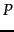) is the fraction of retrieved documents that are relevant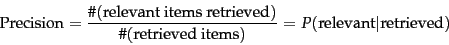(36)

Recall (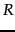) is the fraction of relevant documents that are retrieved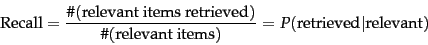(37)

These notions can be made clear by examining the following contingency table: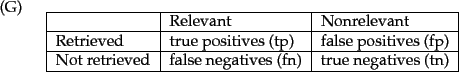Then: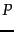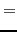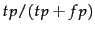(38)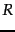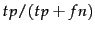(39)

An obvious alternative that may occur to the reader is to judge an information retrieval system by its accuracy , that is, the fraction of its classifications that are correct. In terms of the contingency table above,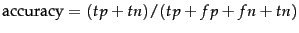. This seems plausible, since there are two actual classes, relevant and nonrelevant, and an information retrieval system can be thought of as a two-class classifier which attempts to label them as such (it retrieves the subset of documents which it believes to be relevant). This is precisely the effectiveness measure often used for evaluating machine learning classification problems.

There is a good reason why accuracy is not an appropriate measure for information retrieval problems. In almost all circumstances, the data is extremely skewed: normally over 99.9% of the documents are in the nonrelevant category. A system tuned to maximize accuracy can appear to perform well by simply deeming all documents nonrelevant to all queries. Even if the system is quite good, trying to label some documents as relevant will almost always lead to a high rate of false positives. However, labeling all documents as nonrelevant is completely unsatisfying to an information retrieval system user. Users are always going to want to see some documents, and can be assumed to have a certain tolerance for seeing some false positives providing that they get some useful information. The measures of precision and recall concentrate the evaluation on the return of true positives, asking what percentage of the relevant documents have been found and how many false positives have also been returned.

The advantage of having the two numbers for precision and recall is that one is more important than the other in many circumstances. Typical web surfers would like every result on the first page to be relevant (high precision) but have not the slightest interest in knowing let alone looking at every document that is relevant. In contrast, various professional searchers such as paralegals and intelligence analysts are very concerned with trying to get as high recall as possible, and will tolerate fairly low precision results in order to get it. Individuals searching their hard disks are also often interested in high recall searches. Nevertheless, the two quantities clearly trade off against one another: you can always get a recall of 1 (but very low precision) by retrieving all documents for all queries! Recall is a non-decreasing function of the number of documents retrieved. On the other hand, in a good system, precision usually decreases as the number of documents retrieved is increased. In general we want to get some amount of recall while tolerating only a certain percentage of false positives.

A single measure that trades off precision versus recall is the F measure , which is the weighted harmonic mean of precision and recall: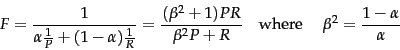(40)

where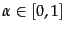and thus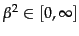. The default balanced F measure equally weights precision and recall, which means making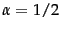or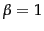. It is commonly written as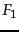, which is short for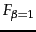, even though the formulation in terms of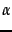more transparently exhibits the F measure as a weighted harmonic mean. When using, the formula on the right simplifies to: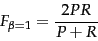(41)

However, using an even weighting is not the only choice. Values of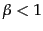emphasize precision, while values of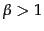emphasize recall. For example, a value of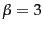or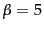might be used if recall is to be emphasized. Recall, precision, and the F measure are inherently measures between 0 and 1, but they are also very commonly written as percentages, on a scale between 0 and 100.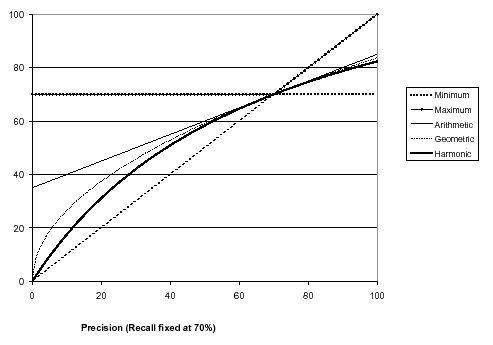Graph comparing the harmonic mean to other means.The graph shows a slice through the calculation of various means of precision and recall for the fixed recall value of 70%. The harmonic mean is always less than either the arithmetic or geometric mean, and often quite close to the minimum of the two numbers. When the precision is also 70%, all the measures coincide.

Why do we use a harmonic mean rather than the simpler average (arithmetic mean)? Recall that we can always get 100% recall by just returning all documents, and therefore we can always get a 50% arithmetic mean by the same process. This strongly suggests that the arithmetic mean is an unsuitable measure to use. In contrast, if we assume that 1 document in 10,000 is relevant to the query, the harmonic mean score of this strategy is 0.02%. The harmonic mean is always less than or equal to the arithmetic mean and the geometric mean. When the values of two numbers differ greatly, the harmonic mean is closer to their minimum than to their arithmetic mean; see Figure 8.1 .

Exercises.

• An IR system returns 8 relevant documents, and 10 nonrelevant documents. There are a total of 20 relevant documents in the collection. What is the precision of the system on this search, and what is its recall?

• The balanced F measure (a.k.a. F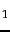) is defined as the harmonic mean of precision and recall. What is the advantage of using the harmonic mean rather than averaging'' (using the arithmetic mean)?

• Derive the equivalence between the two formulas for F measure shown in Equation 40, given that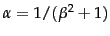.Next: Evaluation of ranked retrieval Up: Evaluation in information retrieval Previous: Standard test collections   Contents   Index